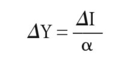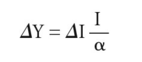# Domar economic growth model

## Domar economic growth model

a theoretical construct that examines the dual role of INVESTMENT in both expanding AGGREGATE DEMAND and the economy's AGGREGATE SUPPLY capacity (POTENTIAL GROSS NATIONAL PRODUCT) over time.

As one of the components of aggregate demand, investment expenditure has the effect of adding to total demand. According to the Keynesian theory of income determination, income will increase until the saving generated by the additional income offsets the higher level of investment (see EQUILIBRIUM LEVEL OF NATIONAL INCOME).

Thus, if we designate the increase in investment as ?I, income (Y) will increase by the amount:where a is the MARGINAL PROPENSITY TO SAVE.

This short-term theory of income determination ignores the other effect of investment spending, i.e. that it adds to productive capacity. This capacity-creating effect is negligible in the short term, but ECONOMIC GROWTH has to do with the long term, and in the long term the role of investment in adding to productive capacity needs to be considered along with its demand-creating effect.

The question posed by Domar was: ‘if investment increases productive capacity and also creates income, at what rate should investment grow in order to make the increase in income equal to that of productive capacity?’ To answer this question, Domar set up an equation, one side of which represented the rate of increase of productive capacity, the other the rate of increase of income, and the solution of which gave the required rate of growth. Let each pound of investment, I , add to productive capacity by the amount £0 per year. For example, if it requires £3,000 of capital to produce £1,000 of output per year, ? will be one-third, or 33% per year. The symbol ? represents the CAPITAL-OUTPUT RATIO, which is the relationship between increments of investment and the resulting increments of output. The productive capacity of the economy has therefore increased by I?, which is the capacity-creating effect of investment and the supply side of our equation.

To utilize this additional capacity, demand must increase by an equal amount. Turning to the demand side of the equation, MULTIPLIER theory tells us that with any given marginal propensity to save, £, an increase in national income is a function not of I but of the increment in investment, ? I, that is, the absolute annual increase in investment. The corresponding absolute annual increase in income is then:where I/£ is the multiplier. To fulfil the condition that income and capacity should increase at the same rate requires that:The left-hand side expression is the annual percentage rate of growth of investment, which, to maintain an assumed full employment with growing productive capacity, must grow at the annual percentage rate £0. Income must also grow at the same annual percentage rate. See SOLOW ECONOMIC GROWTH MODEL, HARROD ECONOMIC GROWTH MODEL.

Site: Follow: Share:
Open / Close